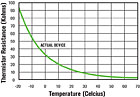Thermistors are one type of temperature sensor used on electronic control systems. Technicians servicing these control systems need to be able to test the operation and accuracy of these sensors. A defective thermistor can easily cause a controller to not operate correctly.

A thermistor is basically a variable resistor whose resistance changes with a change in temperature. There are two basic types of thermistors: negative temperature coefficient (NTC) and positive temperature coefficient (PTC). The resistance of an NTC thermistor will increase with a decrease in temperature. The resistance of a PTC thermistor will decrease with a decrease in temperature.

For example, one type of NTC thermistor can have a resistance of 10,000 ohms at a temperature of 77°F and a resistance of 30,000 ohms at a temperature of 32°. A PTC thermistor can have a resistance of 10,000 ohm at 77° and then a resistance of 5,000 ohms at 32°.

There are many different types of both the NTC and PTC thermistors available. Not all NTC and PTC thermistors will have the same resistance values over a range of temperatures. A control manufacturer or designer will choose a type of NTC or PTC thermistor that works best with their controller. (Figure 1 is an example of a temperature vs. resistance chart of one type of NTC thermistor.)

## TESTING

Testing the operation of these sensors is quite simple: Remove the sensor from the controller and allow the thermistor to approach room temperature. Place an ohmmeter across the leads of the thermistor and record its resistance value. Then place the thermistor in an ice bath. If the thermistor is an NTC type, its resistance will increase; if the thermistor is a PTC type, its resistance will decrease. This test will determine if the thermistor is functioning, but it does not determine if it is accurate.

To test the accuracy of a thermistor, you will need to get the temperature vs. resistance chart from its manufacturer. This chart will state the correct resistance values of the thermistor at varying temperatures. To conduct this test, again place an ohmmeter across the leads of the thermistor and then expose it to a known temperature, such as an ice bath (32°). Compare the measured resistance with the chart’s resistance - the two should be very close. You may want to test the thermistor at one other temperature such as room temperature to be certain the thermistor is responding accurately.

A technician can also test the operation of the electronic control module by replacing the thermistor with a known resistance. For example, suppose a temperature controller has a set point of 40°. At this temperature, the controller’s contacts should be closed. If an NTC thermistor type sensor is used on the controller and the thermistor should have a resistance of 25,000 ohms at 40°, if a technician replaces the thermistor with a fixed 10,000 ohm resistor, the controller will “interrupt” the resistance as a temperature above 40° and should close its contacts.

When replacing a temperature sensor, make sure you use the correct sensor. First, not all temperature sensors are thermistors. There are other types of temperature sensors that can be used on electronic controllers. Secondly, not all thermistors have the same temperature vs. resistance relationship. It is usually best to obtain the correct temperature sensor from the OEM or from your local distributor who carries that manufacturer’s products.

Publication date:11/02/2009